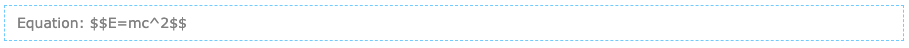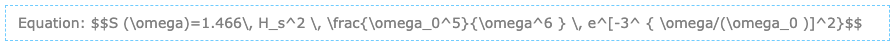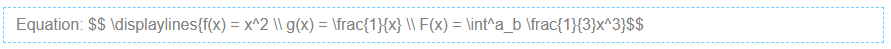# Equations with LaTeX

Mathematical equations may be created using LaTeX notation.

While editing a chapter, select Tools > LaTeX Equation and type in the formula. Upon submitting, you will see a box in the editor that looks like the following:You can continue to edit the formula in the editor (provided that you do not remove the double-\$ symbols). When saved, the example equation will render like this:

$$E=mc^2$$

Here is a more sophisticated example:Which will render like this:

$$S (\omega)=1.466\, H_s^2 \, \frac{\omega_0^5}{\omega^6 } \, e^[-3^ { \omega/(\omega_0 )]^2}$$

For multi-line equations, use a double-slash (\\) to separate each line as follows:$$f(x) = x^2 \\ g(x) = \frac{1}{x} \\ F(x) = \int^a_b \frac{1}{3}x^3$$

If you need assistance writing LaTeX equations, there are many online tools that can help you do this such as the Online LaTeX Equation Editor.

All rendering is performed courtesy of MathJax and CodeDogs.